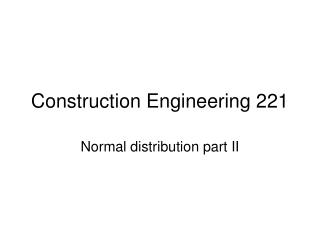Download PresentationConstruction Engineering 221

# Construction Engineering 221 - PowerPoint PPT Presentation

Construction Engineering 221. Normal distribution part II. Normal distribution. Many measurements are normally distributed and can be converted to a standardized distribution in order to use z-tablesI am the owner, or an agent authorized to act on behalf of the owner, of the copyrighted work described.
Download Presentation## Construction Engineering 221

Download Policy: Content on the Website is provided to you AS IS for your information and personal use and may not be sold / licensed / shared on other websites without getting consent from its author.While downloading, if for some reason you are not able to download a presentation, the publisher may have deleted the file from their server.

- - - - - - - - - - - - - - - - - - - - - - - - - - E N D - - - - - - - - - - - - - - - - - - - - - - - - - -
Presentation Transcript
1. Construction Engineering 221 Normal distribution part II

2. Normal distribution • Many measurements are normally distributed and can be converted to a standardized distribution in order to use z-tables • To calculate probabilities, it is typical to pick an acceptable level (say, .05) and calculate probability that z is greater than or less than that value (this will be important in hypothesis testing)

3. Normal distribution • Example: • Concrete cylinder test results (in psi) are 1000, 1200, 1150, 1100, and 1230. You need to have three of six cylinders exceed 1200 psi before you can strip forms. What is the probability that the sixth cylinder will exceed 1200 psi? • First calculate the mean and sd of the sample • Mean = 1136, sd = 90

4. Normal distribution • Z-statistic for the sixth cylinder is • 1200-1136/90 = .711 • Z= .711, P = .26115 • Probability is (1 - .76115), or .2389, 24% chance • must add 50% area from left of the mean and subtract remaining area to find probability

5. Normal distribution • What if the specifications read that the average of six cylinders must be 1200? • 1200 X 6 = 7200 • Σ (1-5) = 5680 • Cylinder 6 must test at 7200-5680 = 1520 for average to be 1200 • Z = 1520-1200/176.5 = 1.81 • A(x) = .46485 • P= 1-(.5 + .46485) = .0352, or 3% chance

6. Normal distribution • What if you asked to change the specification to read the average of the 5 highest of 6 tests (eliminate the outlier of 1000) must equal 1200? • 1200 + 1150 + 1100 + 1230 +X/5 = 1200 • X= 1320 (sixth cylinder must test at 1320 • Z= 1320-1200/83.37 = 1.44 • A(x) = .42507 • P= 1-.92507, or 7.5%

7. Normal Distribution • Is this important? • Assume an 18 million dollar job with a 12 month completion schedule • 1.5 million per month/ 22 workdays per = 68,200 per day • .03 X 68200 = \$2046 • .075 X 68200 = \$5115 • risk savings of over \$3000 for a simple change • Consider you make (50,000 X1.4)/264 or \$265/day

8. Normal distribution • Normal approximation of the Binomial distribution is used when the number of trials is large • Math gets cumbersome • Large sample sizes approach continuous distributions • Use normal approximations when values exceed those included in binomial tables • Use when n*π >5 (or n* (1-π)), or when n>30

9. Normal distribution • Do 6 and 10 on page 82